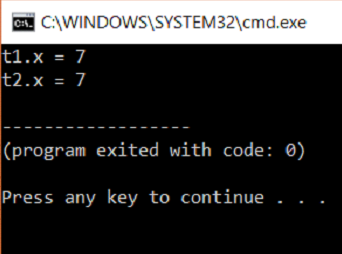AWS Global Infrastructure

### Programming & Frameworks

Topics Covered
• C Programming and Data Structures (17 Blogs)
• Comprehensive Java Course (4 Blogs)
• Java/J2EE and SOA (345 Blogs)
• Spring Framework (8 Blogs)
SEE MORE

# How To Implement Copy Constructor In C++?How To Implement Copy Constructor In C++?

So let us get started with this article on Copy Constructor in C++

A Copy Constructor is a Constructor which initializes an object of a class using another object of the same class.

Syntax:

We have the keyword const because we want to make the value constant and make sure that it is not modified somewhere in the code. Like a default constructor, a copy constructor is also provided by the compiler. This is called Default Copy Constructor. Copy constructors can be made private. We cannot copy the objects of the class when we make the copy constructor private.

Here is an Example Code:

```#include<iostream>
using namespace std;
class test
{
private:
int x;
public:
test(int x1)
{
x = x1;
}
test(const test &t2)
{
x = t2.x;
}
int getX()
{
return x;
}
};
int main()
{
test t1(7); // Normal constructor is called here
test t2 = t1; // Copy constructor is called here
cout << "t1.x = " << t1.getX();
cout << "nt2.x = " << t2.getX();
return 0;
}
```

Output:## Explanation

The above program is a basic demo of a copy constructor. We have a class test, with a private data member of type int called x. Then we have a parameterized constructor, which assigns 7 to the variables x. We have a copy constructor, which instantiates the value of t2 with the value of t1. Address of t2 is sent which holds the value of t1 and is assigned to x. There exist a get function which returns the value of x.

The main function has an object of class test called t1. There is a value associated with this object, this is a parameter. The main function has another object of class test called t2. This is initialized by using the t1 variable and the copy constructor is called here. Lastly, the get function is called with respect to t1 and t2 to get the value of x.

Types

There are two types of the copy constructor.
⦁ Shallow Copy Constructor
⦁ Deep Copy Constructor

## Shallow copy constructor:

A Shallow Copy Constructor is a Default Copy Constructor.

Example:

Two people access a database at the same time and make changes to the values over two different systems. If they make changes to the database then, both these changes will be shown in the database. Both objects will point to the same memory location. This is Shallow Copy Constructor. This mostly happens when we are working with the default copy constructor. Here is an Example Code For: Shallow Copy Constructor:

```#include <iostream>
using namespace std;
class test {
public:
int y, z;
test( int y1,int z1)
{
y = y1;
z = z1;
}
};
int main()
{
test a(7,13);
cout <<"the sum is: "<< a.y+a.z;
return 1;
}
```

## Output:## Explanation:

In the above code, we use a default copy constructor provided by the compiler. Hence it is a Shallow Copy Constructor.

## Deep copy constructor

Deep Copy Constructor is a user-defined copy constructor.
For Example:
When two people must make a presentation and they both copy from the same source, the copies are separate. So, when you make modifications only the other copy is not affected. This is Deep Copy Constructor. Both the objects will be pointing at different memory locations and the changes in one won’t have any effects on the other. The memory is dynamically allocated.

Here is an Example Code For: Deep Copy Constructor:

```#include<iostream>
using namespace std;
class test
{
private:
int x;
public:
test(int x1)
{
x = x1;
}
test(const test &t2)
{
x = t2.x;
}
int getX()
{
return x;
}
};
int main()
{
test t1(7); // Normal constructor is called here
test t2 = t1; // Copy constructor is called here
cout << "t1.x = " << t1.getX();
cout << "nt2.x = " << t2.getX();
return 0;
}
```

Output:## Explanation

This is the same code we have used above, giving similar output. It is a user defined Copy Constructor and hence it is a Deep Copy Constructor.

Thus we have come to an end of this article on ‘Copy Constructor in C++’. If you wish to learn more, check out the Java Training by Edureka, a trusted online learning company. Edureka’s Java J2EE and SOA training and certification course is designed to train you for both core and advanced Java concepts along with various Java frameworks like Hibernate & Spring.

Got a question for us? Please mention it in the comments section of this blog and we will get back to you as soon as possible.REGISTER FOR FREE WEBINARThank you for registering Join Edureka Meetup community for 100+ Free Webinars each month